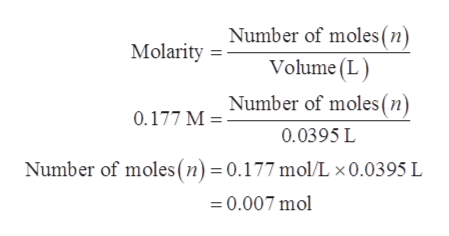# How many moles of HCl are present in 39.5 mL of 0.177 M HCl?

Question
9 views

How many moles of HCl are present in 39.5 mL of 0.177 M HCl?

check_circle

Step 1

Molarity is the described as the number of moles present in 1 L solution. Molarity is expressed in mol/L. The mathematical expression is shown below.

Step 2

Given data:

Step 3

Substitute the given data in equ...help_outlineImage TranscriptioncloseNumber of moles (n) Volume (L Number of moles (n) Molarity 0.177 M = 0.0395 L Number of moles (n) = 0.177 mol/L x0.0395 L 0.007 mol fullscreen

### Want to see the full answer?

See Solution

#### Want to see this answer and more?

Solutions are written by subject experts who are available 24/7. Questions are typically answered within 1 hour.*

See Solution
*Response times may vary by subject and question.
Tagged in

### Physical Chemistry